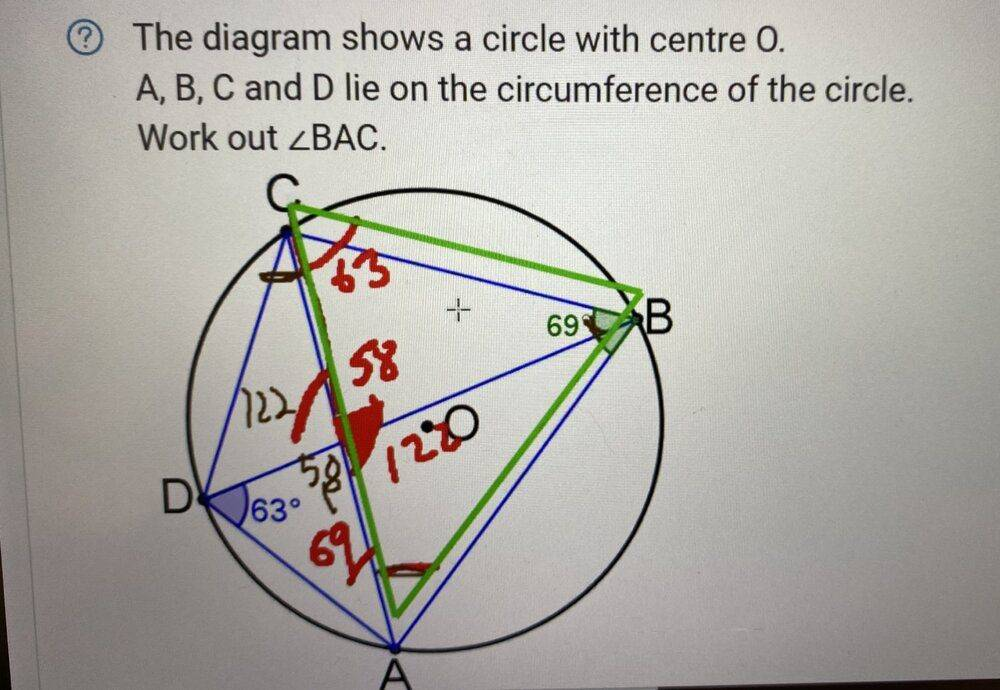# Circle theorem -- Find the angle BAC between these points on the circumference of a circle

Natasha1
Homework Statement:
The diagram shows a circle with centre O.
Relevant Equations:
A, B, C and D lie on the circumference of the circle. Work out angle BAC
Please see attached drawing which I have written my work (in colour).

Despite spending 1h on it I still can’t understand how to find angle BAC.Last edited by a moderator:

Natasha1
I found it! It’s 48 degrees

Quantour
1) For a quadrilateral inscribed in a circle, the sum of opposite angles equals to 180 degrees.
2) Inscribed angle theorem

•Delta2
Homework Helper
I found it! It’s 48 degrees
In that case BD would be parallel to BA which it is plainly not. There is a 48 degree angle where you've marked 58. I'm not sure we can solve for BAC since chord DA's length can be shrunk or stretched without materially affecting the rest of the given geometry. Sum of angles BAC and ABD should be 48 by exterior angle thm.

Last edited:
Homework Helper
Gold Member
2022 Award
I'm not sure we can solve for BAC since chord DA's length can be shrunk or stretched without materially affecting the rest of the given geometry.
Not by moving A because that would change ABC, and if you move D then BAC doesn't change anyway.
In that case BD would be parallel to BA

How do you deduce that? I agree with 48 degrees.

Homework Helper
Maybe I am misinterpreting the marked angles ? Why has the OP marked 58 degrees when the other 2 angles in the triangle are 63 and 69 degrees. I think the confusion arises because I thought (and it looks like the OP also initially thought) that the measure of angle CBD was 69 degrees. But now I see it's CBA which is 69 degrees. Then the 48 degree answer makes sense.

If a person thought CBD was 69 degrees, he/she would also mark CAD as 69 degrees as appears to be the case.

Last edited: